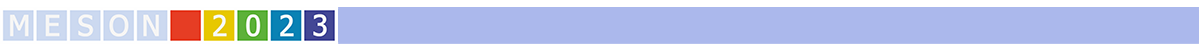#MESON2023

Jun 22 – 27, 2023
Auditorium Maximum
Europe/Warsaw timezone

## Test for non-relativistic QED in decays of Positronium atoms

Jun 23, 2023, 4:00 PM
20m
Medium lecture hall (A) (Auditorium Maximum)

Parallel

### Speaker

Sushil Sharma (Jagiellonian University)

### Description

The study of a bound system of two particles is the most appropriate tool to understand the nature of forces. In the high energy regime, quantum chromodynamics (QCD) is commonly used to understand the forces between quarks carrying a color charge . Moreover, the bound system of heavy quarks ( charm - $c$, bottom - $b$ ) and their anti-quarks ( $\overline{c}$, $\overline{b}$ ), forming a family of heavy mesons called quarkonium (charmonium ($c\bar{c}$), bottomonium ($b\bar{b}$), is a solid basis for the study of non-relativistic QCD (nrQCD) . In the low energy regime, there is a structural analogus to the bound state of the quarkonium and the simplest two-body system consisting only of leptonic objects, the positronium atom (Ps). It consists of an electron bound to its anti-particle positron, describes the interactions of electrically charged particles, and is a sensitive probe of quantum electrodynamics (QED) . Prior to annihilation, $e^{+}$ and $e^{-}$ can also form in one of two states, depending on the total spin number (S) - para-positronium (p-Ps, S=0) and ortho-positronium (o-Ps, S=1). The analogy between positronium and quarkonium can be extended to other phenomena, such as the energy levels and decay rates of Ps atoms, which can be accurately calculated using the formalism of non-relativistic QED (nrQED) . However, the experimental estimate of the o-Ps decay rate is still two orders of magnitude less precise than the theoretically predicted values [5,6].

In this work, we present a method for estimating the o-Ps decay rate in vacuum, which requires simultaneous measurement of o-Ps and p-Ps decays , and finally compare the results with the predictions of QED. For this work, the data were measured with the J- PET, a multiphoton detector suitable for studying the decays of Ps atoms [8,9]. Our goal is to improve the accuracy of the decay rate measurement by an order of magnitude compared to the best measurement to date .

Collaboration J-PET

### Primary author

Sushil Sharma (Jagiellonian University)

### Co-authors

Kamil Dulski (Jagiellonian University) Paweł Moskal (Jagiellonian University)

### Presentation materials

There are no materials yet.